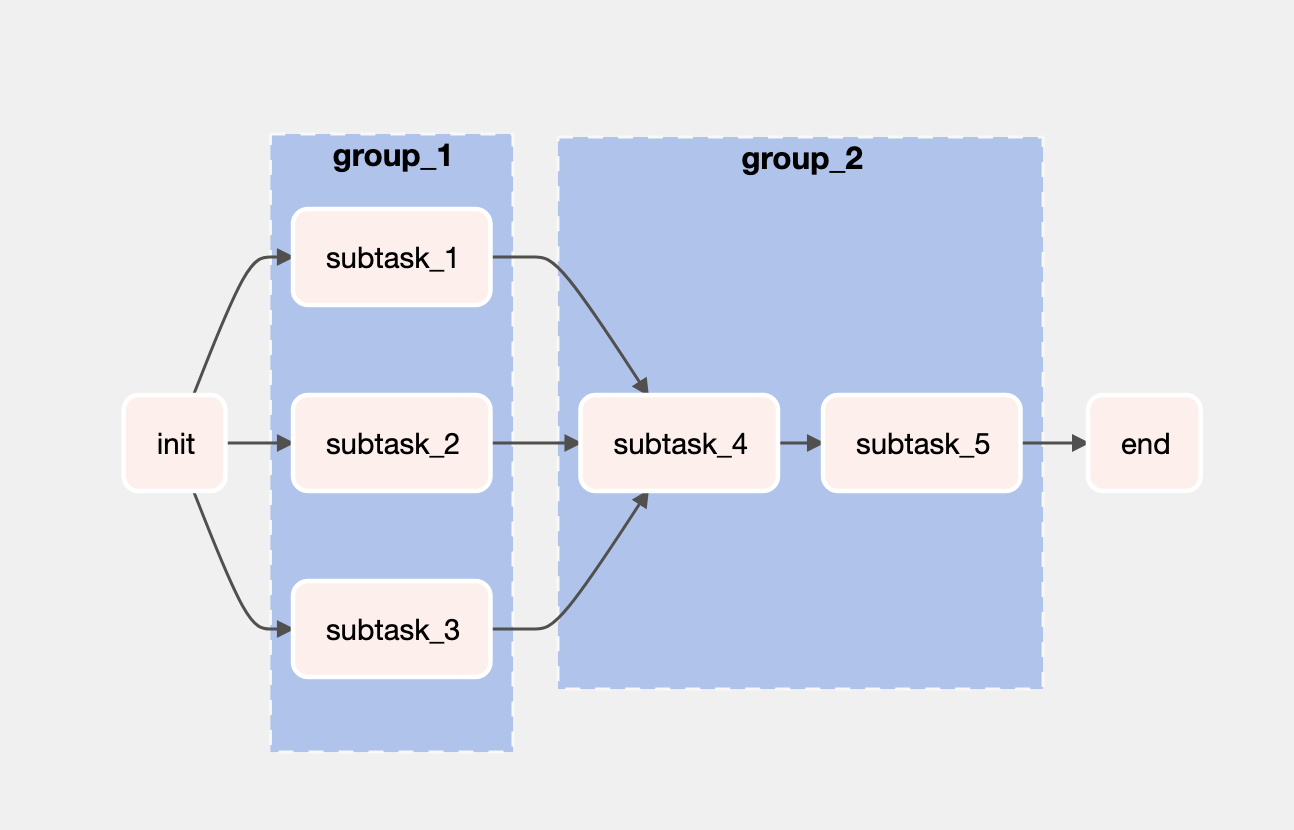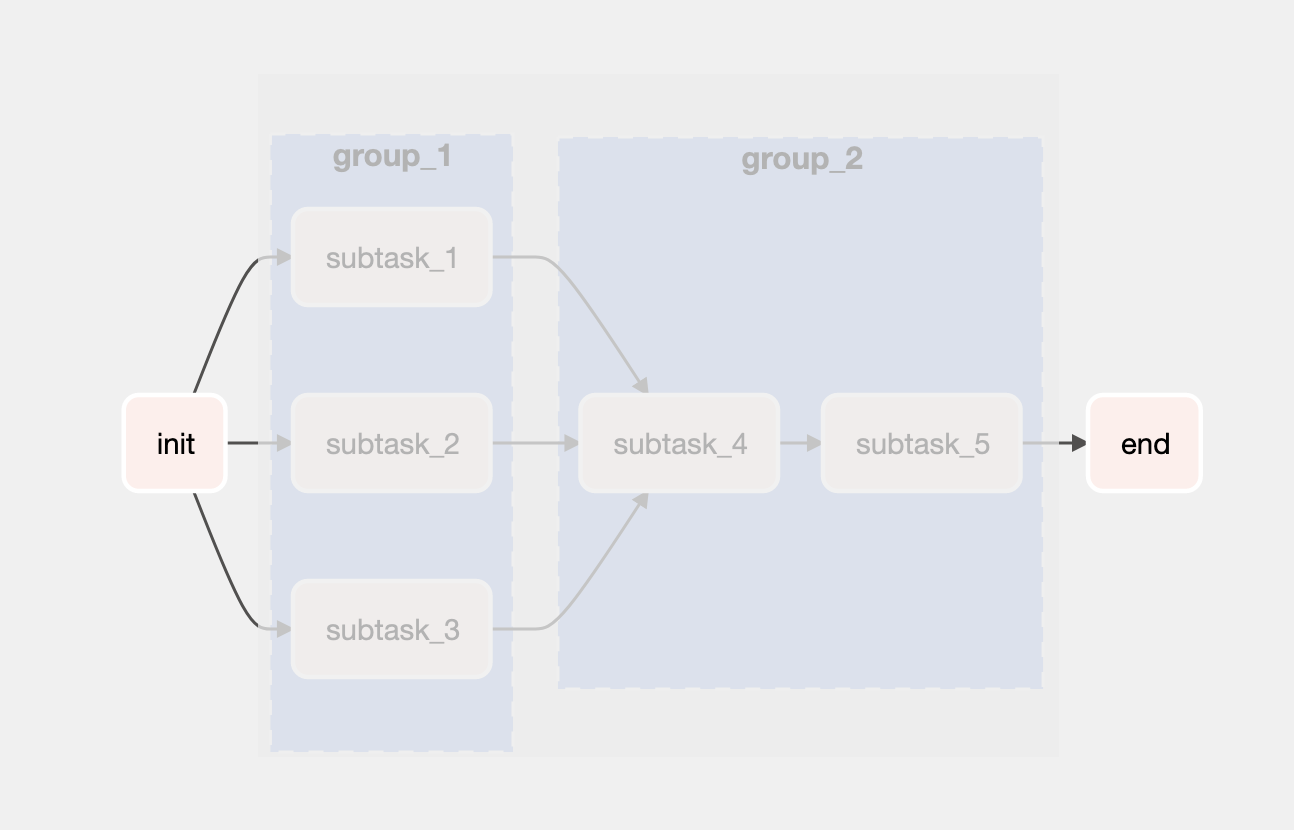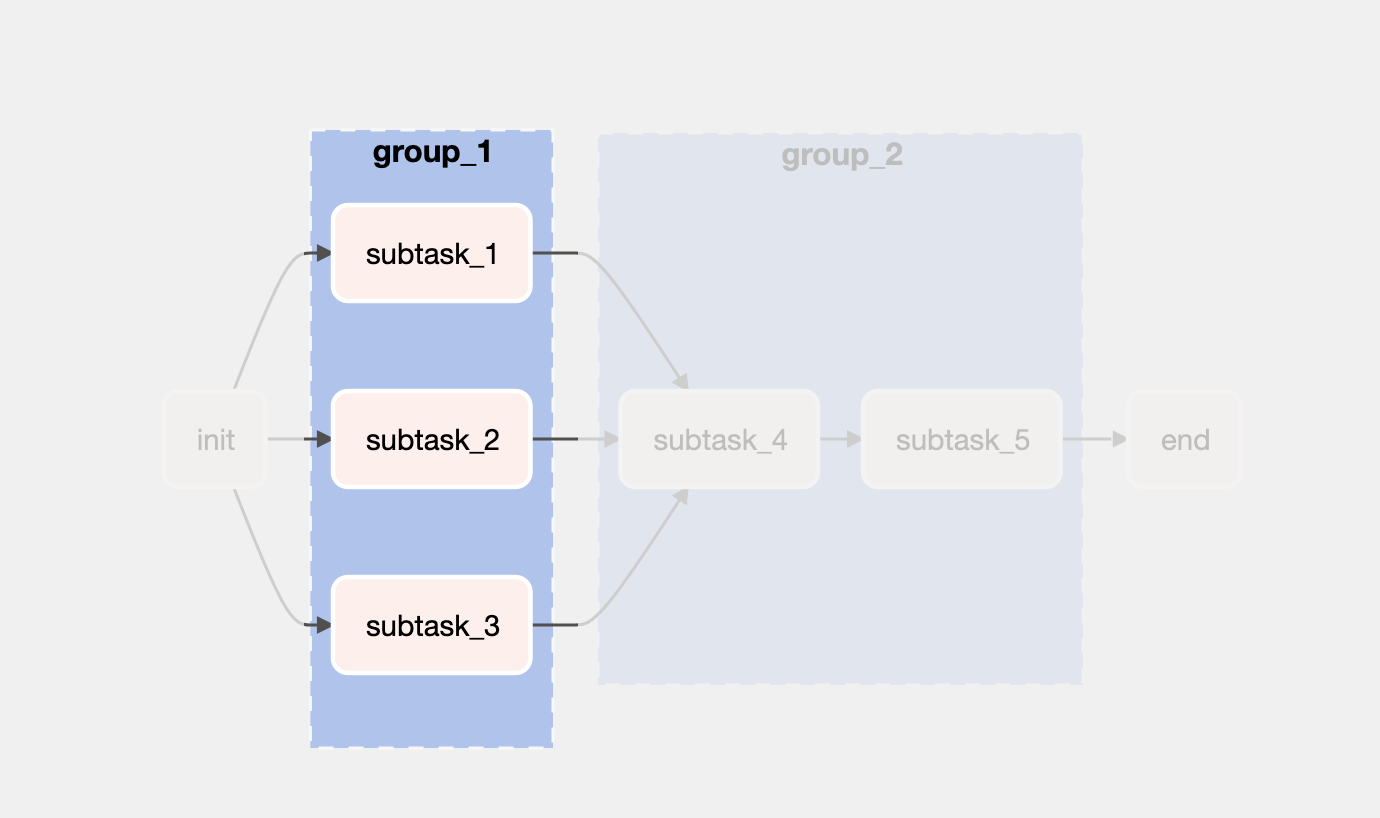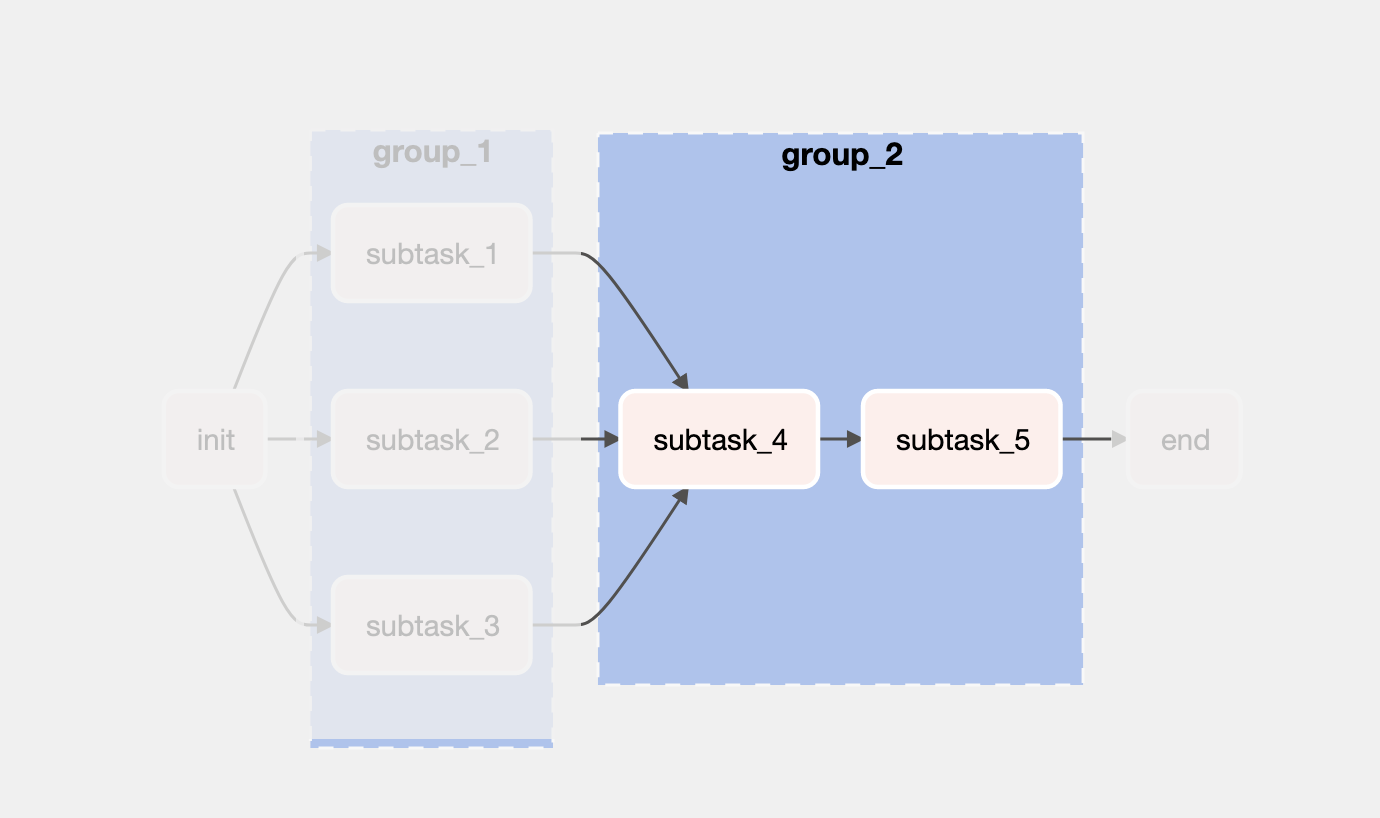## Source code#

The complete code is available here.

## Intro#

Before Task Groups in Airflow 2.0, Subdags were the go-to API to group tasks. With Airflow 2.0, SubDags are being relegated and now replaced with the Task Group feature. The TaskFlow API is simple and allows for a proper code structure, favoring a clear separation of concerns.

What we’re building today is a simple DAG with two groups of tasks, using the `@taskgroup` decorator from the TaskFlow API from Airflow 2. The graph view is:What this pipeline does is different manipulations to a given initial value. The `init()` task instantiates a variable with the value `0`. It then passes to a group of subtasks (`group_1`) that manipulate that initial value. `group_2` will aggregate all the values into one. Finally, the `end()` subtask will print out the final result.

Let’s get started by breaking the pipeline down into parts.

As you can see in the image above, there’s an `init()` and `end()` task. In between, there are two groups of tasks, but let’s start with the first and last task of the pipeline.

### `init()` task and `end()` task#The `init()` task is the starting point for this pipeline - it returns the initial value that will be manipulated throughout the pipeline: 0. The `end()` task will print out all the manipulations in the pipeline, to the console.

Let’s look at the code for the `init()` task:

``````@task
def init():
return 0
``````

That’s it. Now the code for the `end()` task:

``````@task
def end(value):
print(f'this is the end: {value}')
``````

It’s also quite simple to define the flow of the whole pipeline, returned by the function that wraps everything:

``````return end(group_2(group_1(init())))
``````

Looking at the code above it’s possible to see that:

1. The `init()` function “feeds” `group_1`;
2. The result of `group_1` is “sent” to `group_2`;
3. `end()` receives the outcome of `group_2`.

### Task Group #1 (`group_1`)#`group_1` has a set of three tasks that manipulate the original number:

``````# This task group has three subtasks:
# each subtask will perform an operation on the initial value
# this group will return a list with all the values of the subtasks
def group_1(value):

# The @tasks below can be defined outside function `group_1`
# What matters is where they are referenced

# sending this list to `group_2`
``````

The `group_1` function receives the result from the `init()` task. And notice what’s being returned here: a list of the three values. Each of the value stems from `subtask_1`, `subtask_2` and `subtask_3`. This list of values is what’s going to be sent to `group_2`.

### Task Group #2 (`group_2`)#`group_2` is rather simple. It receives the list sent from `group_1` and sums all values (`subtask_4` does it) and then `subtask_5` just multiplies by two the result from task_4:

``````@task_group(group_id='group_2')
def group_2(list):

return sum(values)

return value*2

# task_4 will sum the values of the list sent by group_1
# task_5 will multiply it by two.
That’s it - the next task is the `end()`, and it has been handled before in this post. Again, it’s possible to see the full code here.
If you check the log of the `end()` task (see my previous post to know how to check for task logs), you’ll see the result printed. The final result should be `12`.